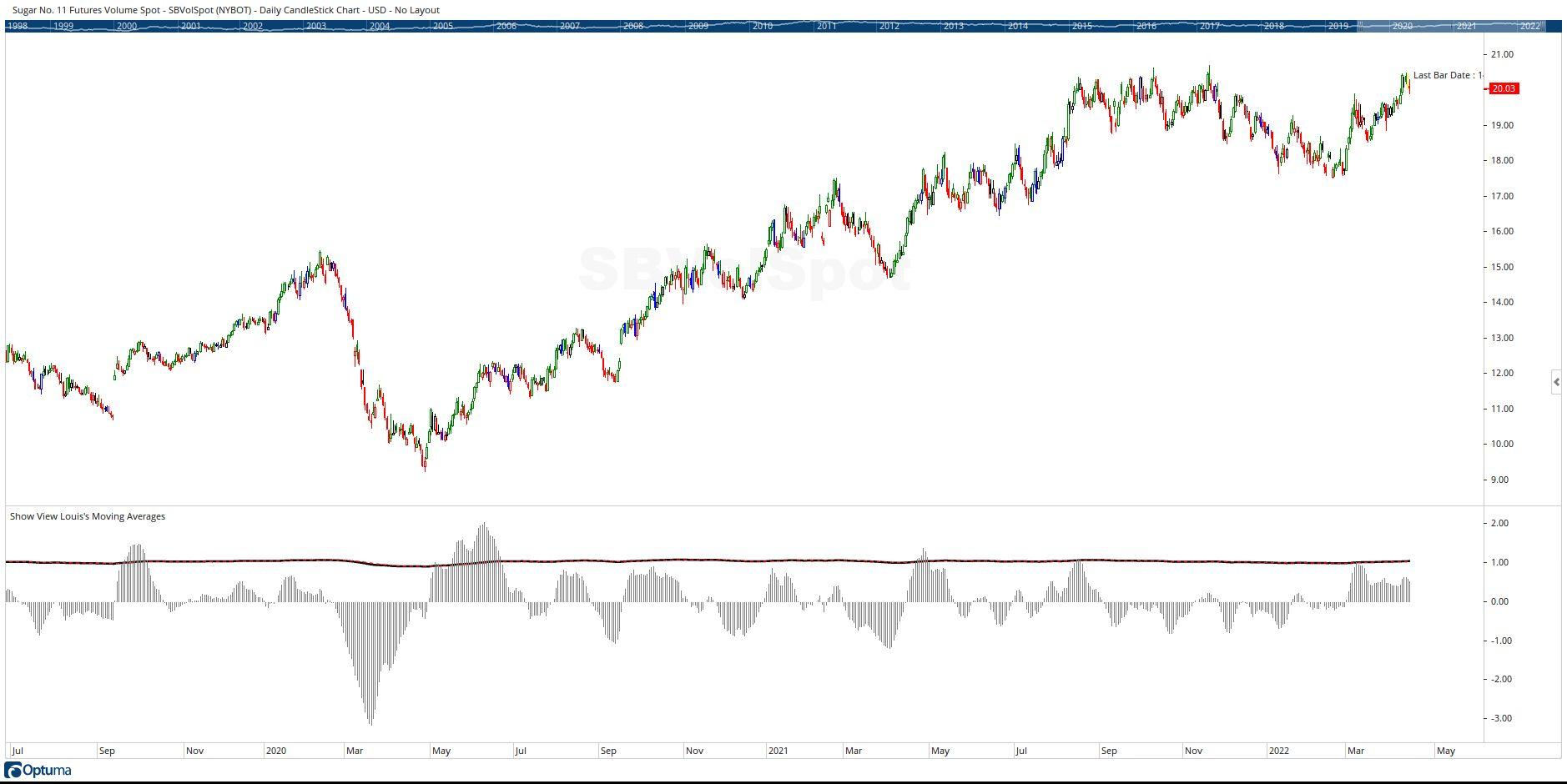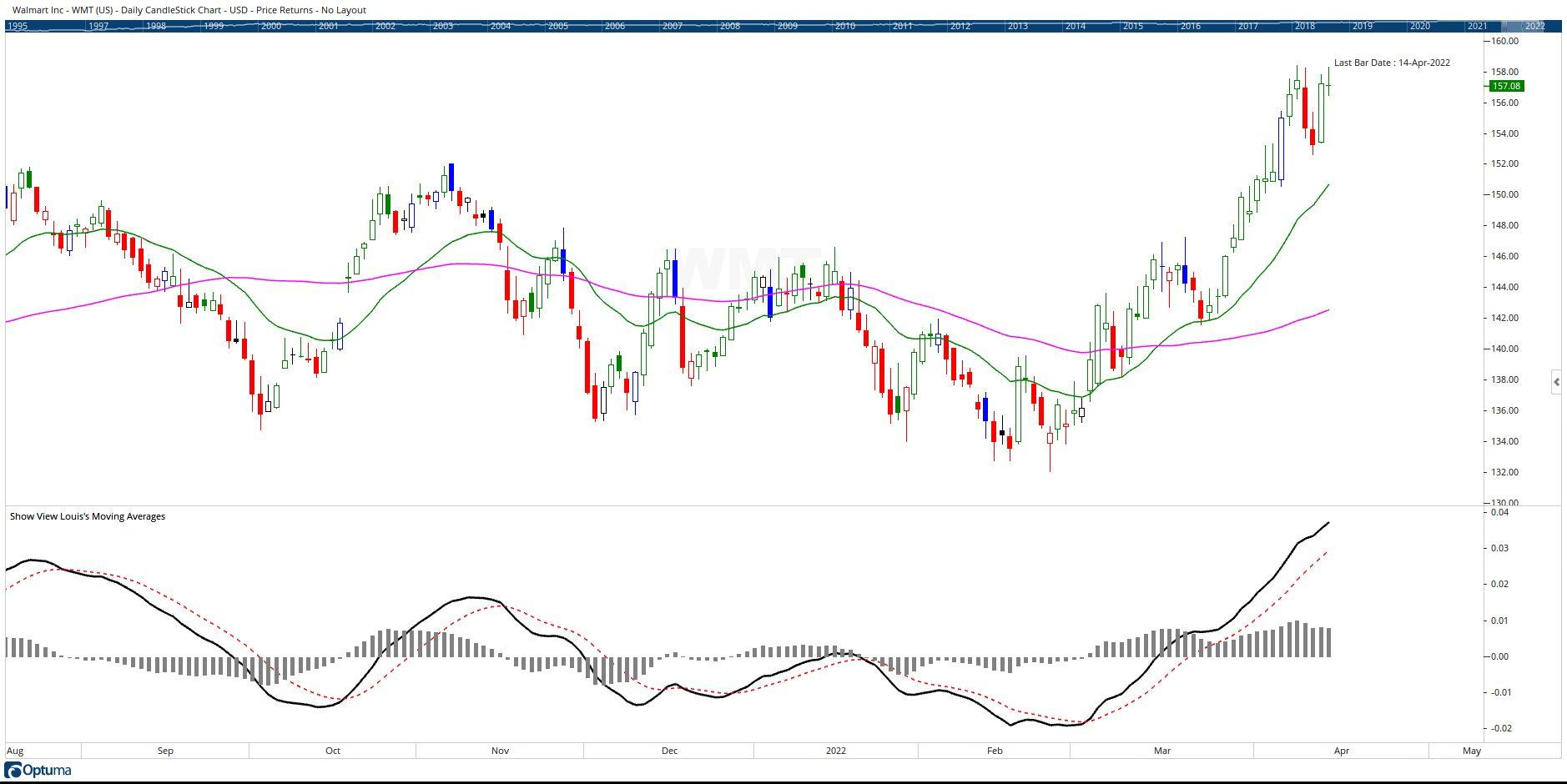Select Page

# Pring’s Trend Deviation Script

Optuma Forums Optuma Scripting Pring’s Trend Deviation Script

Viewing 5 posts - 1 through 5 (of 5 total)
• Author
Posts
• #67949

Hi guys,

Plots 1 and 2 work perfectly – see picture 1. The problem I am having is when trying to display  a histogram, called plot 3, which will subtract plot 2 from plot 1. Picture 2 is what happens. Please advise on how to correct this script so the histogram displays properly.

many thanks!

#67957

Hi Louis,

Your line Plot3 = (Plot1 – Plot2) ; is trying to subtract one array from another array. Plots are arrays and that doesn’t work. You need to do the subtraction on the creation of Plot3:

Plot3 = (MA1 / MA2) – (MA(Plot1, BARS=9, CALC=Close, STYLE=Exponential) );

Note that the values for Plot 3 are very small compared to Plot1 and Plot2, so to see the values all on one view you would need to multiply Plot3 by some factor, say 100,  like this:

Plot3 = ((MA1 / MA2) – (MA(Plot1, BARS=9, CALC=Close, STYLE=Exponential) ) )* 100;

which will produce the result shown in the following screenshot:Cheers

Trevor

#67961

Thanks for letting me know. I want this think to look like a MACD, or PPO, or KST. The only difference in my formula I am doing a division to calculate plot 1 instead of subtraction or addition. Your solution does not allow plots 1 and 2 to be see as they are in picture 1 above. Any ideas?

#67965

Hi Louis,

See if this code results in what you are looking for:

This is what is gives me:Cheers

Trevor

#67993

Hi Trevor. You nailed it! I was trying to multiply plot3, but your approach of subtracting 1 from plot1 was a great idea! Thank you so much.

Viewing 5 posts - 1 through 5 (of 5 total)
• You must be logged in to reply to this topic.